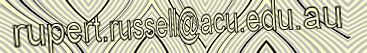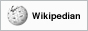Home > Tutorials > Processing > Processing Examples > Spirograph I

# Spirograph I

see example online at openprocessing.org

#### processing code

```// A variation of Spirograph  by Ali Khan, licensed under Creative Commons at OpenProcessing.org.
// http://www.openprocessing.org/visuals/?visualID=17230
// Used to create http://www.redbubble.com/people/rupertrussell/t-shirts/7055494-spirograph-v

SpiroCircle sc;
float r1, incr;
boolean pause;
int count;

float currentMouseX;
float currentMouseY;

void setup() {
size(400,400);
strokeWeight(25);
smooth();

sc = new SpiroCircle();
r1 = 0.0;
incr = 0.05;
pause = false;
}

void draw() {

r1+=incr;
if(r1>width/2)
r1=0.0;

if(keyPressed) {
if(key>='1' && key<='9')
incr=((int) key-'1'+1)/20.0;

if(key==' ')
pause=true;
else
pause=false;
}

if(!pause) {
background(0);

currentMouseX = mouseX;
currentMouseY = mouseY;

if(currentMouseX == 0){
currentMouseX = 300;
}

if(currentMouseY == 0){
currentMouseY = 300;
}

println(str(currentMouseX));

sc.init(width/2, height/2, r1, currentMouseX/2, currentMouseY/2);
sc.drawIterations(1000, incr);
}

/*
if (mousePressed){
count ++;
save("AE_" + str(count));
}
*/

}

class SpiroCircle {

int center_x = 0;
int center_y = 0;
float r1 = 0;
float r2 = 0;
float h = 0;
float x, y, prev_x, prev_y;
int iter;
boolean first;

// constructor
public void SpiroCirle() {
}

public void init(int x, int y, float radius_1, float radius_2, float point_distance) {
center_x = x;
center_y = y;
h = point_distance;
first = true;
}

public void drawIterations(int iter, float incr) {

for(int i=0; i < iter * 1; ++i) {
stroke((sin(PI*i/iter)*255)-5 );
}
}

float x, y;

if(first) {
first = false;
}
else {
strokeWeight(6);
// line(prev_x, prev_y, x,y);
// strokeWeight(17);
point(x,y);
}

prev_x = x;
prev_y = y;
}
}```

code formatter

APA citation:
Russell, R. (2016, July 05, 07:26 am). Spirograph I.
Retrieved June 07, 2023, from http://www.rupert.id.au/tutorials/processing/examples/spirograph/index.php

Last refreshed: June 07 2023. 03:23.04 pmThis work is licensed under a Creative Commons Attribution-NonCommercial-ShareAlike 2.5 License.

271 visits since April 22, 2011